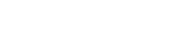# math.acosh

`FLOATmath.acoshFLOATx`

Available inall subroutines.

Computes the inverse hyperbolic cosine of its argument `x`.

## Parameters

`x` - Floating point value representing the area of a hyperbolic sector.

## Return Value

Upon successful completion, this function returns the inverse hyperbolic cosine of `x`.

If `x` is `math.NAN`, a NaN will be returned.

If `x` is +1, +0 will be returned.

If `x` is `math.POS_INFINITY`, `math.POS_INFINITY` will be returned.

If `x` is `math.NEG_INFINITY`, a domain error occurs and a NaN will be returned.

For finite values of `x` < 1, a domain error occurs and a NaN will be returned.

## Errors

If the `x` argument is finite and less than +1.0, or is `math.NEG_INFINITY`, then `fastly.error` will be set to `EDOM`.

## Example

``````declare local var.fo FLOAT;
set var.fo = math.acosh(10);``````

BETA